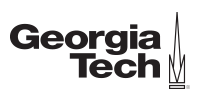Ir al contenido principal

# Learn methods that can solve real-world problemsCertificación Profesional en
Applications of Linear Algebra
GTx

## Lo que aprenderás

• Model and solve real-world problems using Markov chains, determinants, dynamical systems, and Google Page Rank.
• Construct the singular value decomposition (SVD) of a matrix and apply the SVD to estimate the rank and condition number of a matrix, construct a basis for the four fundamental spaces of a matrix, and construct a spectral decomposition of a matrix.
• Apply the iterative Gram Schmidt Process and the QR decomposition to construct an orthogonal basis of a subspace.
• Apply least-squares and multiple regression to construct a linear model from a data set.
• Apply eigenvalues and eigenvectors to solve optimization problems that are subject to distance and orthogonality constraints.

This certificate program will take students through roughly seven weeks of MATH 1554, Linear Algebra, as taught in the School of Mathematics at The Georgia Institute of Technology.

In the first course, you will explore the determinant, which yields two important results. First, you will be able to apply an invertibility criterion for a square matrix that plays a pivotal role in, for example, computer graphics and in other more advanced courses, such as multivariable calculus. The first course then moves on to eigenvalues and eigenvectors. The goal of this part of the course is to decompose the action of a linear transformation that may be visualized. The main applications described here are to discrete dynamical systems, including Markov chains. However, the basic concepts afforded by eigenvectors and eigenvalues are useful throughout industry, science, engineering and mathematics.

In the second course you will explore methods to compute an approximate solution to an inconsistent system of equations that have no solutions. This has a central role in the understanding of current data science applications. The second course then turns to symmetric matrices. They arise often in applications of the singular value decomposition, which is another tool often found in data science and machine learning.

Capacitación de la mano de expertos
2 cursos de capacitación
A tu ritmo
Avanza a tu ritmo
2 meses
5 - 6 horas por semana
398 US\$
Para obtener la experiencia completa del programa

1. ## Certificación Profesional en Applications of Linear Algebra de GTx

2. 5–6 horas por semana durante 3 semanas

This course takes you through roughly three weeks of MATH 1554, Linear Algebra, as taught in the School of Mathematics at The Georgia Institute of Technology.

3. 5–6 horas por semana durante 3 semanas

This course takes you through roughly five weeks of MATH 1554, Linear Algebra, as taught in the School of Mathematics at The Georgia Institute of Technology.

• Linear algebra is a foundational topic for data science and engineering occupations. Employment in mathematics occupations is expected to grow 27 percent from 2019 to 2029 (source: Occupational Outlook Handbook)

## Conoce a tu instructor de The Georgia Institute of Technology (GTx)

Greg Mayer
Academic Professional in the School of Mathematics
Georgia Tech (Georgia Institute of Technology)

Expertos de GTx comprometidos con el aprendizaje en línea

## Inscríbete ahora

398 US\$
2 cursos en 2 meses